Updating search results...

# 14 Results

View
Selected filters:
• parallelogramsUnrestricted Use
CC BY
Rating
0.0 stars

Family facing 6th Grade math unit focusing on area and surface area.

Subject:
Geometry
Mathematics
Material Type:
Unit of Study
Provider:
Illustrative Mathematics
05/11/2020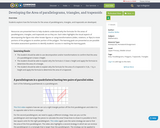Conditional Remix & Share Permitted
CC BY-NC-SA
Rating
0.0 stars

Students explore how the formulas for the areas of parallelograms, triangles, and trapezoids are developed.&nbsp;

Subject:
Mathematics
Material Type:
Activity/Lab
Homework/Assignment
Author:
Heather Bolles
05/13/2020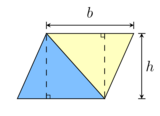Conditional Remix & Share Permitted
CC BY-SA
Rating
0.0 stars

Measurement in Grade 7 sees us move from simply finding the perimeter and area of a common object, such as a rectangle or triangle, and move into complex shapes that may involve a combination of different shapes or circles.&nbsp;This unit reviews the basics of finding the area of rectangles, triangles, and parallelograms. It concludes with some real-life problems to which these concepts can be applied.This unit is designed for the Alberta Grade 7 Curriculum.

Subject:
Measurement and Data
Material Type:
Activity/Lab
Homework/Assignment
Module
Unit of Study
Author:
10/10/2022Conditional Remix & Share Permitted
CC BY-NC
Rating
0.0 stars
Rating
0.0 stars
Subject:
Mathematics
Material Type:
Full Course
Provider:
Pearson
10/06/2016Conditional Remix & Share Permitted
CC BY-NC
Rating
0.0 stars

Surface Area and Volume

Type of Unit: Conceptual

Prior Knowledge

Students should be able to:

Identify rectangles, parallelograms, trapezoids, and triangles and their bases and heights.
Identify cubes, rectangular prisms, and pyramids and their faces, edges, and vertices.
Understand that area of a 2-D figure is a measure of the figure's surface and that it is measured in square units.
Understand volume of a 3-D figure is a measure of the space the figure occupies and is measured in cubic units.

Lesson Flow

The unit begins with an exploratory lesson about the volumes of containers. Then in Lessons 2–5, students investigate areas of 2-D figures. To find the area of a parallelogram, students consider how it can be rearranged to form a rectangle. To find the area of a trapezoid, students think about how two copies of the trapezoid can be put together to form a parallelogram. To find the area of a triangle, students consider how two copies of the triangle can be put together to form a parallelogram. By sketching and analyzing several parallelograms, trapezoids, and triangles, students develop area formulas for these figures. Students then find areas of composite figures by decomposing them into familiar figures. In the last lesson on area, students estimate the area of an irregular figure by overlaying it with a grid. In Lesson 6, the focus shifts to 3-D figures. Students build rectangular prisms from unit cubes and develop a formula for finding the volume of any rectangular prism. In Lesson 7, students analyze and create nets for prisms. In Lesson 8, students compare a cube to a square pyramid with the same base and height as the cube. They consider the number of faces, edges, and vertices, as well as the surface area and volume. In Lesson 9, students use their knowledge of volume, area, and linear measurements to solve a packing problem.

Subject:
Geometry
Mathematics
Material Type:
Unit of Study
Provider:
PearsonConditional Remix & Share Permitted
CC BY-NC
Rating
0.0 stars

Lesson OverviewStudents find the area of a parallelogram by rearranging it to form a rectangle. They find the area of a trapezoid by putting together two copies of it to form a parallelogram. By doing these activities and by analyzing the dimensions and areas of several examples of each figure, students develop and understand area formulas for parallelograms and trapezoids.Key ConceptsA parallelogram is a quadrilateral with two pairs of parallel sides. The base of a parallelogram can be any of the four sides. The height is the perpendicular distance from the base to the opposite side.A trapezoid is a quadrilateral with exactly one pair of parallel sides. The bases of a trapezoid are the parallel sides. The height is the perpendicular distance between the bases.You can cut a parallelogram into two pieces and reassemble them to form a rectangle. Because the area does not change, the area of the rectangle is the same as the area of the parallelogram. This gives the parallelogram area formula A = bh.You can put two identical trapezoids together to form a parallelogram with the same height as the trapezoid and a base length equal to the sum of the base lengths of the trapezoid. The area of the parallelogram is (b1 + b2)h, so the area of the trapezoid is one-half of this area. Thus, the trapezoid area formula is A = 12(b1 + b2)h.Goals and Learning ObjectivesDevelop and explore the formula for the area of a parallelogram.Develop and explore the formula for the area of a trapezoid.

Subject:
Geometry
Material Type:
Lesson Plan
09/21/2015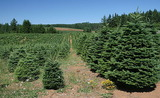Conditional Remix & Share Permitted
CC BY-NC
Rating
0.0 stars

Lesson OverviewStudents find the area of a triangle by putting together a triangle and a copy of the triangle to form a parallelogram with the same base and height as the triangle. Students also create several examples of triangles and look for relationships among the base, height, and area measures. These activities lead students to develop and understand a formula for the area of a triangle.Key ConceptsTo find the area of a triangle, you must know the length of a base and the corresponding height. The base of a triangle can be any of the three sides. The height is the perpendicular distance from the vertex opposite the base to the line containing the base. The height can be found inside or outside the triangle, or it can be the length of one of the sides.You can put together a triangle and a copy of the triangle to form a parallelogram with the same base and height as the triangle. The area of the original triangle is half of the area of the parallelogram. Because the area formula for a parallelogram is A = bh, the area formula for a triangle is A = 12bh.Goals and Learning ObjectivesDevelop and explore the formula for the area of a triangle.

Subject:
Geometry
Material Type:
Lesson Plan
09/21/2015Conditional Remix & Share Permitted
CC BY-NC
Rating
0.0 stars

Four full-year digital course, built from the ground up and fully-aligned to the Common Core State Standards, for 7th grade Mathematics. Created using research-based approaches to teaching and learning, the Open Access Common Core Course for Mathematics&nbsp;is designed with student-centered learning in mind, including activities for students to develop valuable 21st century skills and academic mindset.

Subject:
Mathematics
Material Type:
Full Course
Provider:
Pearson
10/06/2016Conditional Remix & Share Permitted
CC BY-NC
Rating
0.0 stars

Constructions and Angles

Unit Overview

Type of Unit: Concept

Prior Knowledge

Students should be able to:

Use a protractor and ruler.
Identify different types of triangles and quadrilaterals and their characteristics.

Lesson Flow

After an initial exploratory lesson involving a paper folding activity that gets students thinking in general about angles and figures in a context, the unit is divided into two concept development sections. The first section focuses on types of angles—adjacent, supplementary, complementary, and vertical—and how they are manifested in quadrilaterals. The second section looks at triangles and their properties, including the angle sum, and how this affects other figures.

In the first set of conceptual lessons, students explore different types of angles and where the types of angles appear in quadrilaterals. Students fold paper and observe the angles formed, draw given angles, and explore interactive sketches that test many cases. Students use a protractor and ruler to draw parallelograms with given properties. They explore sketches of parallelograms with specific properties, such as perpendicular diagonals. After concluding the investigation of the angle types, students move on to the next set.

In the second set of conceptual development lessons, students focus on triangles. Students again fold paper to create figures and certain angles, such as complementary angles.

Students draw, using a protractor and ruler, other triangles with given properties. Students then explore triangles with certain known and unknown elements, such as the number of given sides and angles. This process starts with paper folding and drawing and continues with exploration of interactive sketches. Students draw conclusions about which cases allow 0, 1, 2, or an infinite number of triangles. In the course of the exploration, students discover that the sum of the measure of the interior angles of a triangle is 180°. They also learn that the sum of the measures of the interior angles of a quadrilateral is 360°. They explore other polygons to find their angle sum and determine if there is a relationship to angle sum of triangles. The exploration concludes with finding the measure of the interior angles of regular polygons and speculating about how this relates to a circle.

Lastly, students solve equations to find unknown angle measures. Using their previous experience, students find the remaining angle measures in a parallelogram when only one angle measure is given. Students also play a game similar to 20 Questions to identify types of triangles and quadrilaterals. Having completed the remaining lessons, students have a four-day Gallery to explore a variety of problems.

The unit ends with a unit assessment.

Subject:
Geometry
Mathematics
Material Type:
Unit of Study
Provider:
Pearson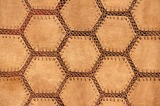Conditional Remix & Share Permitted
CC BY-NC
Rating
0.0 stars

Students learn more about the characteristics of parallelograms by folding paper and measuring the angles in a parallelogram. Students use a ruler and protractor to draw parallelograms with given properties. Then, students use a ruler and protractor to draw a rectangle.Key ConceptsOpposite angles of a parallelogram are congruent.Consecutive angles of a parallelogram are supplementary.Diagonals of a parallelogram bisect each other.Diagonals of a rectangle are congruent.Goals and Learning ObjectivesAccess prior knowledge of parallelograms.Understand that the sum of angle measures in any quadrilateral is 360&deg;.Understand the relationship of the angles and diagonals in a parallelogram.Understand the relationship of the angles and diagonals in a rectangle.

Subject:
Geometry
Material Type:
Lesson Plan
09/21/2015Conditional Remix & Share Permitted
CC BY-NC
Rating
0.0 stars

Students learn how the diagonals of a rhombus are related. They use interactive sketches to learn about the properties of the angles and diagonals of squares, rectangles, rhombuses, parallelograms, and other quadrilaterals.Key ConceptsThe sum of the measures of the angles of all quadrilaterals is 360&deg;.The alternate angles (nonadjacent angles) of rhombuses and parallelograms have the same measure.The measure of the angles of rectangles and squares is 90&deg;.The consecutive angles of parallelograms and rhombuses are supplementary. This applies to squares and rectangles as well.The diagonals of a parallelogram bisect each other.The diagonals of a rectangle are congruent and bisect each other.The diagonals of a rhombus bisect each other and are perpendicular.Goals and Learning ObjectivesMeasure the angles formed by the intersection of the diagonals of a rhombus.Explore the relationships of the angles of different squares, rectangles, rhombuses, parallelograms, and other quadrilaterals.Explore the relationships of the diagonals of different squares, rectangles, rhombuses, parallelograms, and other quadrilaterals.

Subject:
Geometry
Material Type:
Lesson Plan
09/21/2015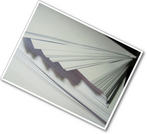Conditional Remix & Share Permitted
CC BY-NC
Rating
0.0 stars

Gallery OverviewAllow students who have a clear understanding of the content thus far in the unit to work on Gallery problems of their choosing. You can then use this time to provide additional help to students who need review of the unit&rsquo;s concepts or to assist students who may have fallen behind on work.Problem DescriptionsParallelogram to CubeStudents have a chance to review angle measurements in a parallelogram. Building the cube helps students see the transition from two-dimensional shapes and their relationship to three-dimensional figures.QuadrilateralsStudents investigate the possible quadrilaterals that can be made from any four given side lengths, focusing on those that can&rsquo;t make a quadrilateral. Students also look at possible parallelograms with two sides given and possible rhombuses with four sides given.DiagonalsStudents further investigate diagonals in quadrilaterals. If the diagonals are perpendicular, is the figure a rhombus?TrapezoidsHow many right angles can a trapezoid have? How many congruent angles or congruent sides can it have? Can its diagonals be perpendicular or congruent? Students investigate possible trapezoids.More AnglesStudents explore three intersecting lines and the combinations of angles.Diagonals and AnglesThe sides of a parallelogram are extended beyond the vertices, and students explore which angles are congruent and which are supplementary. Students also explore the effect diagonals have on interior angles.Exterior AnglesStudents&nbsp; explore the sum of exterior angles for several polygons and speculate about the results.Angles and SidesStudents explore the relationship between angles and sides in a triangle and discover that the longest side is opposite the largest angle, and the shortest side is opposite the smallest angle (and congruent sides are opposite congruent angles).Ratios and AnglesStudents explore the ratios of the legs of a right triangle to the angles in the triangle. Students see that there is a unique ratio for each angle, and vice versa. This is an informal look at trigonometry.Find the AngleStudents solve equations to find angle measures in polygons.TessellationsStudents explore quadrilateral tessellations and why they tessellate. Students also explore tessellations of pentagons and other polygons.

Subject:
Geometry
Material Type:
Lesson Plan
09/21/2015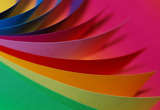Conditional Remix & Share Permitted
CC BY-NC
Rating
0.0 stars

Students discuss what they know about shapes and their characteristics through a paper-folding activity that results in a parallelogram.Key ConceptsQuadrilaterals and triangles are classified by their different characteristics; the types of angles and sides define the shapes. While students are familiar with some of the characteristics of these shapes, they begin to explore other aspects of theses figures. Students review what they know about these shapes so far.Goals and Learning ObjectivesReview characteristics that describe quadrilaterals and triangles.Discuss what students know about these shapes.Explore other aspects of these shapes.

Subject:
Geometry
Material Type:
Lesson Plan
09/21/2015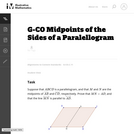Unrestricted Use
CC BY
Rating
0.0 stars

This is a reasonably direct task aimed at having students use previously-derived results to learn new facts about parallelograms, as opposed to deriving them from first principles. The solution provided (among other possibilities) uses the SAS trial congruence theorem, and the fact that opposite sides of parallelograms are congruent.

Subject:
Geometry
Mathematics
Material Type:
Activity/Lab
Provider:
Illustrative Mathematics
Provider Set:
Illustrative Mathematics
Author:
Illustrative Mathematics